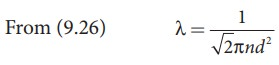Home | | Physics 11th std | Solved Example Problems for Mean Free Path

# Solved Example Problems for Mean Free Path

Physics : Kinetic Theory of Gases - Solved Example Problems for Mean Free Path

### EXAMPLE 9.6

An oxygen molecule is travelling in air at 300 K and 1 atm, and the diameter of oxygen molecule is 1.2 × 10−10m. Calculate the mean free path of oxygen molecule.

### SolutionWe have to find the number density n By using ideal gas lawTags : Kinetic Theory of Gases | Physics , 11th Physics : UNIT 9 : Kinetic Theory of Gases
Study Material, Lecturing Notes, Assignment, Reference, Wiki description explanation, brief detail
11th Physics : UNIT 9 : Kinetic Theory of Gases : Solved Example Problems for Mean Free Path | Kinetic Theory of Gases | Physics